# Physics - Collision in 3 dimensions

Modeling collisions involves a lot of assumptions and approximations, also the concept of an 'impulse' is not always intuitively obvious. These issues are all discussed on the page above this and it may be worth reading that page before this.

One difficult bit is understanding how to work with objects that have both rotation and linear motion. The main method we will use to do this involves the following stages.

1. Determine the collision point and the normal direction at this point.
2. Calculate the minimum impulse to prevent the objects intersecting, or to
reverse the approach velocity, depending on the coefficient of friction.
3. Calculate the effect of this impulse on the rotation and linear motion
separately.

The results, for this 3D case, are summarised as follows:

General case
impulse J =
 -(1+e) (va-vb)•n + (ra×n)•ωa - (rb×n)•ωb1/ma+1/mb+(ra×n)•([Ia]-1(ra×n))+(rb×n)•([Ib]-1(rb×n))
Final velocity of
object a=vaf =
vai - J/Ma
Final velocity of
object b=vbf =
vbi + J/Mb
Final angular velocity of object a=waf = wai - [Ia]-1(J × ra)
Final angular velocity of object b=wbf = wbi + [Ib]-1(J × rb)

## Directions

When we work out these equations manually its easy enough to work out the direction of the impulse and therefore the sign in the above terms but when this is part of a computer program its important to understand the conventions that we are assuming and to get the signs right.

In the following diagrams the arrows indicate the positive direction, not the absolute velocity, which may well be negative.

before collision at collision after collision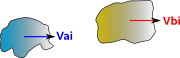vai > vbi   vaf <= vbf

In this case vai > vbi before the collision and vaf <= vbf after. So the impulse subtracts momentum from object a and adds it to object b so we get:

vaf = vai - J/Ma
vbf =vbi + J/Mb

Now we reverse the order of object a and object b:

before collision at collision after collision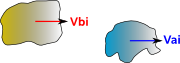vbi > vai   vbf <= vaf

In this case vbi > vai before the collision and vbf <= vaf after. So the impulse subtracts momentum from object b and adds it to object a so we get:

vaf = vai + J/Ma
vbf =vbi - J/Mb

Now it seems a bit messy to get the program to test which is the highest velocity to decide which equations to use so its probably easier to use a negative J to indicate the second case. If you do this be aware that a negative J does not mean its pulling rather than compressing, it just means the objects have collided in the other direction.

## Rotation Directions

Things are more complicated with rotations in that angular momentum must be measured relative to some given point. So if object 'a' is rotating clockwise about its centre of mass it may well impart anticlockwise momentum to object 'b' about its own centre of mass. However this would not necessarily change the angular momentum of the total system, even for a closed system, due to the linear motion of the different centres of mass.

before collision at collision after collision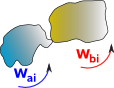ωbiai   ωbf <= ωaf

velocity of collision point on object 'a' =

vpa = vca + ωa×(r-pa)

velocity of collision point on object 'b' =

vpb = vcb + ωb×(r-pb)

## Derivation

As with the 1D case we can start with the definition of the coefficient of restitution which is the ratio of converging and diverging velocities.

However, in this case, the velocities are not the velocities of the centre of mass, they are the velocities of points (in absolute coordinates) on the solid bodies, these are the points on the bodies that actually collide.

e = (vaf- vbf) / (vai - vbi)

 where: other definitions symbol description type units vcaf final velocity of collision point on object a vector m/s vcai initial velocity of collision point on object a vector m/s vcbf final velocity of collision point on object b vector m/s vcbi initial velocity of collision point on object b vector m/s e coefficient of restitution which depends on the nature of the two colliding materials scalar none

let Δvca = vcai - vcaf

This is the change in velocity of the collision point on body 'a' due to the impulse.

and Δvcb = vcbi - vcbf

This is the change in velocity of the collision point on body 'b' due to the impulse.

so,

e * (vcai - vcbi)= -(vcaf- vcbf)

(e+1) * (vcai - vcbi)= -(vcaf- vcbf)+(vcai - vcbi)

(e+1) * (vcai - vcbi)= Δvca + Δvcb

The way that Δvca and Δvcb are related to impulse (J) is explained on this page, from that page we get:

ΔVp = J/M + ([I]-1(J × r)) × r

 where: other definitions symbol description type units Vp linear velocity of a particle on a solid body (vector) vector m/s J impulse vector I inertia scalar such that torque = I * alpha (only true when object is symmetrical) scalar [I] inertia tensor 3×3 matrix m mass scalar kg • • = dot product × × = cross product r position of particle relative to centre of mass - Note: this is in absolute coordinates, not local body coordinates, so this will be a function of time as the body rotates. vector

So we get:

(e+1) * (vai - vbi)= J/Ma +([Ia]-1(J × ra)) x ra + J/Mb +([Ib]-1(J × rb)) x rb

We want to solve this for J, to do this we need to separate out the J terms on the right hand side. The direction of the impulse will depend on the nature of the material colliding and its friction coefficient. If the friction is high then the impulse will be in the direction of approach of the colliding points, if the friction is low then the impulse will be perpendicular to the colliding surfaces, there is no impulse parallel to the surface because they can slide in that direction. So splitting the impulse into its magnitude and direction can allow friction to be taken into account and allow the impulse magnitude to be taken out of the right hand side as a common term.

J = n*|J|

 where: other definitions symbol description type units n normal vector which gives the direction of the impulse. vector J impulse vector vector |J| impulse magnitude scalar

Assuming that there is such a solution we need to make

([Ia]-1(J × ra)) × ra = |J| * ([Ia]-1(n × ra)) × ra

So the result is:

|J| = (e+1) * (vai - vbi) / (1/Ma +n•([Ia]-1(n × ra)) x ra + 1/Mb +n•([Ib]-1(n × rb)) × rb)

## Code

```/**
This function calulates the velocities after a 3D collision vaf, vbf, waf and wbf from information about the colliding bodies
@param double e coefficient of restitution which depends on the nature of the two colliding materials
@param double ma total mass of body a
@param double mb total mass of body b
@param matrix Ia inertia tensor for body a in absolute coordinates (if this is known in local body coordinates it must
be converted before this is called).
@param matrix Ib inertia tensor for body b in absolute coordinates (if this is known in local body coordinates it must
be converted before this is called).
@param vector ra position of collision point relative to centre of mass of body a in absolute coordinates (if this is
known in local body coordinates it must be converted before this is called).
@param vector rb position of collision point relative to centre of mass of body b in absolute coordinates (if this is
known in local body coordinates it must be converted before this is called).
@param vector n normal to collision point, the line along which the impulse acts.
@param vector vai initial velocity of centre of mass on object a
@param vector vbi initial velocity of centre of mass on object b
@param vector wai initial angular velocity of object a
@param vector wbi initial angular velocity of object b
@param vector vaf final velocity of centre of mass on object a
@param vector vbf final velocity of centre of mass on object a
@param vector waf final angular velocity of object a
@param vector wbf final angular velocity of object b
*/
CollisionResponce(double e,double ma,double mb,matrix Ia,matrix Ib,vector ra,vector rb,vector n,
vector vai, vector vbi, vector wai, vector wbi, vector vaf, vector vbf, vector waf, vector wbf) {
matrix IaInverse = Ia.inverse();
vector normal = n.normalise();
vector angularVelChangea  = normal.copy(); // start calculating the change in abgular rotation of a
angularVelChangea.cross(ra);
IaInverse.transform(angularVelChangea);
vector vaLinDueToR = angularVelChangea.copy().cross(ra);  // calculate the linear velocity of collision point on a due to rotation of a
double scalar = 1/ma + vaLinDueToR.dot(normal);
matrix IbInverse = Ib.inverse();
vector angularVelChangeb = normal.copy(); // start calculating the change in abgular rotation of b
angularVelChangeb.cross(rb);
IbInverse.transform(angularVelChangeb);
vector vbLinDueToR = angularVelChangeb.copy().cross(rb);  // calculate the linear velocity of collision point on b due to rotation of b
scalar += 1/mb + vbLinDueToR.dot(normal);
double Jmod = (e+1)*(vai-vbi).magnitude()/scalar;
vector J = normal.mul(Jmod);
vaf = vai - J.mul(1/ma);
vbf = vbi - J.mul(1/mb);
waf = wai - angularVelChangea;
wbf = wbi - angularVelChangeb;
}```

## Alternative method using matrices

It seems like a good idea to express all terms using the same type of algebra, it is not possible to express inertia tensor purely in terms of vectors or quaternions so can we calculate purely in terms of matrices?

The relationship between impulse J and the change in velocity of the point where J is applied is derived here giving:

Change in linear velocity of a point on a solid body due to J=

J/M + ([I]-1(J × r)) × r

 -ixx*rz*rz + ixz*rx*rz -ixx*ry*ry + ixy*rx*ry + 1/M -ixy*rz*rz + ixz*ry*rz +ixx*ry*rx - ixy*rx*rx ixy*rz*ry - ixz*ry*ry + ixx*rz*rx - ixz*rx*rx -iyx*rz*rz + iyz*rx*rz -iyx*ry*ry - iyy*rx*ry -iyy*rz*rz + iyz*ry*rz + iyx*ry*rx - iyy*rx*rx + 1/M iyy*rz*ry - iyz*ry*ry + iyx*rz*rx - iyz*rx*rx -izx*rz*rz + izz*rx*rz -izx*ry*ry + izy*rx*ry -izy*rz*rz + izz*ry*rz + izx*ry*rx + izy*rx*rx izy*rz*ry - izz*ry*ry + izx*rz*rx - izz*rx*rx + 1/M
 Jx Jy Jz

Where;

 where: other definitions symbol description type units ixx to izz elements of inverse inertia tensor (in absolute coordinates) rx,ry and rz point at which impulse is applied relative to centre of mass (in absolute coordinates)

So we now include the effect of J on the relative velocities of bodies A and B:

Change in relative linear velocity of collision point due to J=

(e+1) * (vai - vbi)=

 -iaxx*raz*raz + iaxz*rax*raz -iaxx*ray*ray + iaxy*rax*ray + 1/Ma -ibxx*rbz*rbz + ibxz*rbx*rbz -ibxx*rby*rby + ibxy*rbx*rby + 1/Mb -iaxy*raz*raz + iaxz*ray*raz +iaxx*ray*rax - iaxy*rax*rax -ibxy*rbz*rbz + ibxz*rby*rbz +ibxx*rby*rbx - ibxy*rbx*rbx iaxy*raz*ray - iaxz*ray*ray + iaxx*raz*rax - iaxz*rax*rax + ibxy*rbz*rby - ibxz*rby*rby + ibxx*rbz*rbx - ibxz*rbx*rbx -iayx*raz*raz + iayz*rax*raz -iayx*ray*ray - iayy*rax*ray -ibyx*rbz*rbz + ibyz*rbx*rbz -ibyx*rby*rby - ibyy*rbx*rby -iayy*raz*raz + iayz*ray*raz + iayx*ray*rax - iayy*rax*rax + 1/Ma - ibyy*rbz*rbz + ibyz*rby*rbz + ibyx*rby*rbx - ibyy*rbx*rbx + 1/Mb iayy*raz*ray - iayz*ray*ray + iayx*raz*rax - iayz*rax*rax + ibyy*rbz*rby - ibyz*rby*rby + ibyx*rbz*rbx - ibyz*rbx*rbx -iazx*raz*raz + iazz*rax*raz -iazx*ray*ray + iazy*rax*ray -ibzx*rbz*rbz + ibzz*rbx*rbz -ibzx*rby*rby + ibzy*rbx*rby -iazy*raz*raz + iazz*ray*raz + iazx*ray*rax + iazy*rax*rax -ibzy*rbz*rbz + ibzz*rby*rbz + ibzx*rby*rbx + ibzy*rbx*rbx iazy*raz*ray - iazz*ray*ray + iazx*raz*rax - iazz*rax*rax + 1/Ma + ibzy*rbz*rby - ibzz*rby*rby + ibzx*rbz*rbx - ibzz*rbx*rbx + 1/Mb
 Jx Jy Jz

Therefore we need to invert this matrix to get an expression for J in terms of the approch velocity, can anyone help me with this?

## Solution using impulse

impulse transferred between objects= [NEMa]* (vaf - vai)= -[NEMb]*(vbf - vbi)

where:

• [NEMa] = Newton-Euler Matrixfor object a , this is a 6x6 element matrix.
• [NEMb] = Newton-Euler Matrix for object b, this is a 6x6 element matrix.
• vaf= final velocity for object a, this is a vector of dimension 6 containing both the linear and rotational components in all 3 dimensions. (see here for explanation)
• vai= initial velocity for object a, this is a vector of dimension 6 containing both the linear and rotational components in all 3 dimensions.
• vbf= final velocity for object b, this is a vector of dimension 6 containing both the linear and rotational components in all 3 dimensions.
• vbi= Initial velocity for object b, this is a vector of dimension 6 containing both the linear and rotational components in all 3 dimensions.

Note: I am assuming that in the elastic case, that only the linear part of the impulse is transferred between the objects. The external torque is therefore always zero, so the above equation holds true. In the inelastic case there may be an rotational impulse transferred?

The only situation that I can think of where an elastic rotational impulse might occur is the collision of two rotating masses on the same screw thread. Can anyone help me clarify this? Do we need to consider rotational impulse component in the collision of free floating solid objects?

### perfectly inelastic collision

First take the case of perfectly inelastic collisions (where the objects stick together after collision) and their final velocity is equal.

So,

vaf = vbf (this is not quite true because when the objects stick together they will start orbiting around each other, but they will have the same average velocity, which is the velocity of the common centre of mass)

[NEMa]* (vaf - vai)= -[NEMb]*(vbf - vbi)

so substitutingvaf for vbf gives:

[NEMa]* (vaf - vai)= [NEMb]*(-vaf+vbi)

we want to solve to find the value for vaf, when multiplying matrix an vector, multiplication is distributive over addition so,

[NEMa]* vaf - [NEMa]*vai=[NEMb]*vbi- [NEMb]*vaf

We can rearrange these vectors to give:

[NEMa]* vaf + [NEMb]*vaf = [NEMb]*vbi + [NEMa]*vai

I don't know enough about matrix algebra to proceed further here so I'll expand out the terms:

 iaxx iaxy iaxz 0 -haz hay iayx iayy iayz haz 0 -hax iazx iazy iazz -hay hax 0 0 haz -hay ma 0 0 -haz 0 hax 0 ma 0 hay -hax 0 0 0 ma
 waxf wayf wazf vaxf vayf vazf
+
 ibxx ibxy ibxz 0 -hbz hby ibyx ibyy ibyz hbz 0 -hbx ibzx ibzy ibzz -hby hbx 0 0 hbz -hby mb 0 0 -hbz 0 hbx 0 mb 0 hby -hbx 0 0 0 mb
 waxf wayf wazf vaxf vayf vazf
=
 iaxx iaxy iaxz 0 -haz hay iayx iayy iayz haz 0 -hax iazx iazy iazz -hay hax 0 0 haz -hay ma 0 0 -haz 0 hax 0 ma 0 hay -hax 0 0 0 ma
 waxi wayi wazi vaxi vayi vazi
+
 ibxx ibxy ibxz 0 -hbz hby ibyx ibyy ibyz hbz 0 -hbx ibzx ibzy ibzz -hby hx 0 0 hbz -hby mb 0 0 -hbz 0 hbx 0 mb 0 hby -hbx 0 0 0 mb
 wbxi wbyi wbzi vbxi vbyi vbzi

Hmmm I'm not sure that helps! But perhaps there is a way forward, perhaps we could replace the two matrices on the left of the equation with a single matrix which represents the inertia/mass of the combined objects. This also gets round the earlier problem that the two objects orbit round each other, so have a different combined inertia/mass matrix anyway. So,

[NEMcombined]* vaf = [NEMb]*vbi + [NEMa]*vai

so the solution is:

vaf = vbf =[NEMcombined]inverse * ( [NEMb]*vbi + [NEMa]*vai )

The value of the impulse is:

impulse = [NEMa]* (vaf - vai)

impulse = [NEMa]* ([NEMcombined]inverse* ( [NEMb]*vbi + [NEMa]*vai ) - vai)

### perfectly elastic collision

In this case assume that the equation for impulse is the same as inelastic case (but its value is twice because the objects separate at the same rate that they approach).

I don't think this is strictly the case as, in the 3D case without friction then the impulse should be normal to the collision surface, and the rotational impulse should be zero. Can anyone suggest how to calculate the value of this impulse?

impulse = 2 [NEMa]* ([NEMcombined]inverse * ( [NEMb]*vbi + [NEMa]*vai ) - vai)

Final velocity = initial velocity + impulse

vaf = vai + 2 [NEMa]* ([NEMcombined]inverse * ( [NEMb]*vbi + [NEMa]*vai ) - vai)

### Solution using consevation of momentum and energy

By conservation of linear momentum:* [,,] +* [,,] =* [,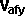,] +* [,,]

By conservation of angular momentum: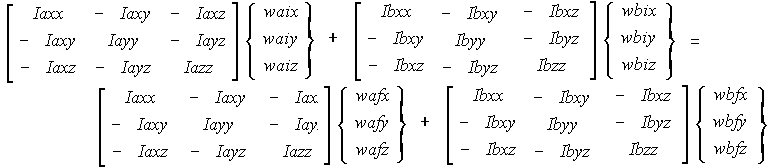where all the rotations in the above equation are about the same point, as explained in the 2D case. If we want to measure the rotations about the objects own centre of mass, then we need to apply the parallel axis theorem, which will add another term to the above equation.

By conservation of energy*+*+*+ Ixx sqr wx + Iyy sqr wy + Izz sqr wz - 2Ixy wx wy - 2Ixz wx wz - 2Iyz wy wz +*+*+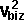*+ Ixx sqr wx + Iyy sqr wy + Izz sqr wz - 2Ixy wx wy - 2Ixz wx wz - 2Iyz wy wz =*+*+*+ Ixx sqr wx + Iyy sqr wy + Izz sqr wz - 2Ixy wx wy - 2Ixz wx wz - 2Iyz wy wz +*+*+*+ Ixx sqr wx + Iyy sqr wy + Izz sqr wz - 2Ixy wx wy - 2Ixz wx wz - 2Iyz wy wz

This gives 7 equations with 12 unknowns so we need extra in formation to solve them:

• velocity of a :,and• velocity of b :,and• rotation of a about x axis: wax
• rotation of a about y axis: way
• rotation of a about z axis: waz
• rotation of b about x axis: wbx
• rotation of b about y axis: wby
• rotation of b about z axis: wbz

So there is insufficient information here to solve these equations so we need to add additional information, such as any constraints that apply, or apply 'angle of incidence = angle of reflection'.

Even if we have enough information to solve in a general case the equations are very complicated to solve for the general case. Specially since we are not usually working in the frame-of-reference of the individual shapes, so in general the I(Inertia) terms will be a function of the angles of the object as it rotates, this will make the equations very complicated. So we may need to take a different approach, as follows: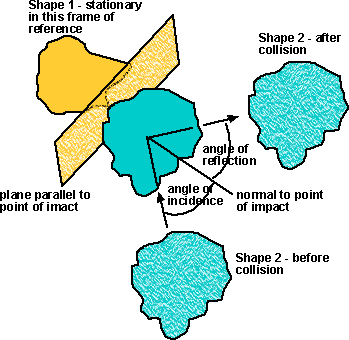Assume we move to the frame of reference of shape 1, therefore shape 1 will always appear stationary in this frame. The movement of shape 2 will be easier to calculate in this frame, for example, if we assume that the impulse is in the direction of the normal only (no sliding). Therefore its motion parallel to the plane will be constant and its motion normal to the plane will be reversed during the collision. There may be energy transferred between the linear and angular motion, depending on the relative positions of the centre-of-mass of shape 2 and the point of collision, but as a first approximation it may be possible to ignore that?

We still have to calculate how the movement of the frame-of-reference has changed in the collision, but this should be relatively easy using the conservation of momentum.

So how to move to the frame-of-reference of shape 1? All we have to do is move shape 2 under shape 1 in the scene graph, not forgetting to translate all the parameters associated with the shape.So the transform [T8] gives the position of shape 2 relative to shape 1.

where in this case:

[T8] = [T7][T3][inverse T2][inverse T5]

We must not forget to transform all the kinematics and dynamics parameters for shape 2 also.

## Rotation Component for 3d example above

If we assume that both objects are traveling in free space (no external torque), then they collide, then they travel again in free space.

As described above, the initial rotations are known, and we want to determine the final rotations. At the collision, energy may be transferred between the colliding objects, and between linear and rotational energies.

The conservation laws of momentum and energy above are not enough to determine the final velocities. We need to take into account other factors determined by the geometry of the objects.

One factor is that the objects can only rotate about certain axes (see no torque). If the object is a perfect sphere (or at least its inertia terms are equal in all directions). Then the object can rotate in any direction, but at least we can use its symmetry to simplify the equations. If the objects are not symmetrical, then they can only rotate about certain axes which will limit the possible outcomes.

Another factor is the position of the point of impact, relative to the centre of masses of the two objects.

If this is too complicated then you might want to consider a numerical approach to collisions.

 metadata block see also: Correspondence about this page Book Shop - Further reading. Where I can, I have put links to Amazon for books that are relevant to the subject, click on the appropriate country flag to get more details of the book or to buy it from them. Commercial Software Shop Where I can, I have put links to Amazon for commercial software, not directly related to the software project, but related to the subject being discussed, click on the appropriate country flag to get more details of the software or to buy it from them.

This site may have errors. Don't use for critical systems.

Copyright (c) 1998-2020 Martin John Baker - All rights reserved - privacy policy.